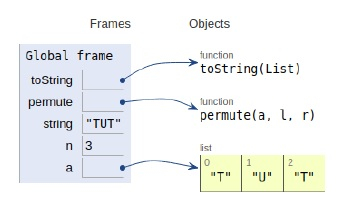# Python Program to print all permutations of a given string

Problem statement − We are given a string we need to display all the possible permutations of the string.

Now let’s observe the solution in the implementation below −

## Example

Live Demo

# conversion
def toString(List):
return ''.join(List)
# permutations
def permute(a, l, r):
if l == r:
print (toString(a))
else:
for i in range(l, r + 1):
a[l], a[i] = a[i], a[l]
permute(a, l + 1, r)
a[l], a[i] = a[i], a[l] # backtracking
# main
string = "TUT"
n = len(string)
a = list(string)
print("The possible permutations are:",end="\n")
permute(a, 0, n-1)

## Output

The possible permutations are:
TUT
TTU
UTT
UTT
TUT
TTUAll the variables are declared in the local scope and their references are seen in the figure above.

## Conclusion

In this article, we have learned about how we can make a Python Program to print all permutations of a given string.